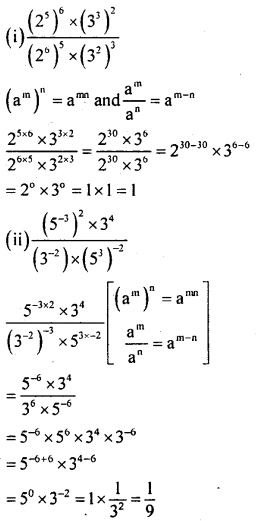# KSEEB Solutions for Class 8 Maths Chapter 10 Exponents Ex 10.4

Students can Download Maths Chapter 10 Exponents Ex 10.4 Questions and Answers, Notes Pdf, KSEEB Solutions for Class 8 Maths helps you to revise the complete Karnataka State Board Syllabus and score more marks in your examinations.

## Karnataka Board Class 8 Maths Chapter 10 Exponents Ex 10.4

Question 1.
Simplify
(i) $$\frac{\left(2^{5}\right)^{6} \times\left(3^{3}\right)^{2}}{\left(2^{6}\right)^{5} \times\left(3^{2}\right)^{3}}$$
(ii) $$\frac{\left(5^{-3}\right)^{2} \times 3^{4}}{\left(3^{-2}\right)^{-3} \times\left(5^{3}\right)^{-2}}$$Question 2.
Can you find two integers m and n such that 2m+“ = 2m”?
m + n = mn
2 + 2 = 2 x 2
4 = 4
∴ m = 2 & n = 2

Question 3.
If (2m)4 = 46 find the value of m.
m =$\frac { 12 }{ 4 }$